﻿ Empty Hole Entropy and Black Hole Entropy in GravitationPublications are Open
Access in this journal
Article Versions
Export Article
• Normal Style
• MLA Style
• APA Style
• Chicago Style
Original Article
Open Access Peer-reviewed

### Empty Hole Entropy and Black Hole Entropy in Gravitation

XiaoLin Li
International Journal of Physics. 2018, 6(5), 155-160. DOI: 10.12691/ijp-6-5-3
Received August 07, 2018; Revised October 03, 2018; Accepted October 12, 2018

### Abstract

In the projection gravitation theory, the center of a gravitational source is an empty hole, not black hole. The gravitation can be understood as a new kind of Lorentz symmetry breaking. Multiple empty holes can merge together to form a large empty hole. So the empty holes form a thermodynamic system. So the empty hole has thermodynamic properties, such as entropy and temperature. We discuss two models of empty holes merging. The first model derives out a result that empty hole entropy is proportional to the radius of the empty hole. The second model can simply derive out the formula of black hole entropy in General Relativity. The black hole entropy is just a special case in empty hole. Two models get the same result that the temperature of the empty hole is inversely proportional to its radius. The empty hole has a new statistical distribution mode, that is the empty hole distribution. In the new projection gravitation, the Planck energy and the Planck length have a new and different physical meaning. The two constants represent a special kind of association relationship that has not yet been understood by humans.

### 1. Introduction

In General Relativity, Bekenstein and Hawking put forward and developed the theory of black hole entropy 2, 3. But the theory of black hole entropy has been debated. The derivation of black hole entropy formula is very complicated and has many doubtful points.

In the previous paper, the author presents a new theory of projection gravitation. The gravitational effect can be seen as a projection effect. In projection gravitation, the center of a gravitational source is an empty hole, not black hole. The empty hole in projection gravitation is very similar to the black holes in General Relativity. But they differ greatly also. The black hole in General Relativity has the entropy and temperature. So, in the projection gravitation, does the empty hole also have entropy and temperature? If so, what is the property of the entropy and temperature? What are the similarities and differences between the empty hole entropy and the black hole entropy? What are the similarities and differences between the empty hole temperature and the black hole temperature? These are the questions that we're going to discuss next.

### 2. Empty Hole in Projection Gravitation

In the previous paper, the author proposes a new gravitation theory. The gravitation is a projection force from 4-dimensional space into 3-dimensional space. The particles have a property of speed symmetry in 4-dimensional space. The Lorentz symmetry in 3-dimensional space is just a projection of the speed symmetry in 4-dimensional space. The projection action not only produces the results of Lorentz symmetry, but also produces an additional projection effect. The additional projection effect is just the gravitation effect. Gravitation is not a real force.

The projection has a force factor. The formula of force factor is (2.1). The force factor is a length constant. M is the rest mass of the gravitation source.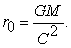(2.1)

In a gravity field, the motion properties of a particle are determined entirely by the force factor. The motion equation of a particle in gravity field is equation (2.2).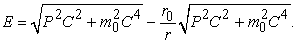(2.2)

In (2.2), E is the total energy of the particle. The second item is the energy of the particle. The third item is the gravitational potential energy of particle. The gravitational potential energy is negative. The distance between the particle and the gravitational center is larger, the gravitational potential energy of the particle is smaller. The force factor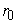uniquely identifies the nature of the gravity field.

We simplify (2.2), get (2.3).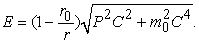(2.3)

Because the energy of a particle cannot be negative, and cannot be zero. So, the distance between the particle and the gravitational center can always be larger than, and cannot be equal with, and cannot be smaller than. This leads to a result that there exists a space area in the gravity field. There is no particle in this space area. Particles will never be able to enter this space area. This space area is a empty hole. The radius of empty hole is just the force factor.

So, the gravitational force can also be seen as an effect of empty hole. Because there is a gravitational source, an empty hole appears in space. So the space symmetry is compromised by the empty hole.

The Lorentz symmetry is actually a projection symmetry. The projection symmetry is a symmetry on the whole space. If there does not exist gravitational source, the particle is free, and its energy is (2.4). (2.4) is a mathematics expression of Lorentz symmetry.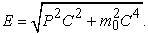(2.4)

We change the form of (2.4). That is (2.5). From (2.5), we can see more clearly the correlation between Lorentz symmetry and space symmetry. Because space has symmetry, so the Lorentz symmetry can be established.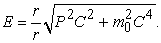(2.5)

Because the empty hole breaks the space symmetry, this will lead to Lorentz symmetry breaking. So, the gravitational effect can be seen as an effect of Lorentz symmetry breaking. The mathematical expression of Lorentz symmetry breaking is formula (2.6). (2.6) is same with (2.3). It's just that the expression form has changed. But (2.6) is more capable of expressing the essence of Lorentz symmetry breaking. But the Lorentz symmetry breaking is not changing the light speed. The light speed is invariant. The breaking is the space symmetry.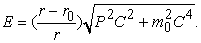(2.6)

This way of looking at gravitation is better suited to quantum mechanics. The gravitation force can appear as symmetry breaking effect in equations of quantum mechanics. So, the gravitation force can be more easily quantized.

Because the gravitation can be seen as Lorentz symmetry breaking, so gravitation force is completely different with electromagnetic force, strong force, weak force. Gravitation force is not a real force. There not have gravition.

### 2. Empty Hole Merging

The empty hole in the projection gravitation is similar to the black hole in the General Relativity. But there is a big difference. The similarity is that both are attracted particles to close. In General Relativity, however, particles can fall into the black hole. But in the projection gravitation, particles can not fall into empty hole. Particles can only infinity close to the empty hole. The minimum distance between the particle and the empty hole is the radius of empty hole.

So, the process of black holes merging in General Relativity corresponds to the process of empty holes merging in projection gravitation. To the process of empty hole merging, in extreme and ideal conditions, two empty holes can only be close to the distance. So the process of empty holes merging is actually the process of empty holes stacking. Many small empty holes stacked together, viewed from afar, can be considered equivalent to a large empty hole.

So, empty holes are not really merged into one empty hole. The process of empty holes merging is only an equivalent approach. This is exactly the same as mass synthesis in gravity. Observing from afar, it is a big hole. But in close observation, there are actually a lot of small holes stacked together. This empty holes stack is represented by Figure 1.

Please pay attention, the empty hole stacking is completely different from regular ball stacking. The empty hole merging is not the combination of volume, but is combination of radius. It is addition of empty hole radius, not is addition of empty hole volume. It is very special. Please reader distinguishes it carefully. This is very important.

So, the process of empty holes merging, as a combination of spherical shells, is easier to understand. Such as figure 2. Figure 2 is only to explain more vividly. But empty hole merging is not actually combination of spherical shells. Please note that empty hole merging is actually addition of radius. Its physical view is the rest mass synthesis of gravitational source.

In the process of empty hole merging, the radius of empty hole may be different. To a large empty hole combined by many small empty holes, we can make an idealized simplification and get an average radius of small empty hole. We assume that each small empty hole has same radius. This is equivalent to that each small empty hole has same rest mass. Different large empty hole just contains different numbers of small empty hole. This is equivalent to the particle processing of the small empty hole. So, a large empty hole is a thermodynamic system, and each small hole is a thermodynamic particle.

We use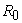to identify the average radius of small empty hole. So, the average rest mass of small empty hole is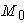.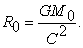(3.1)

We use r to identify the radius of the merged large empty hole. Different large empty hole has different radius r. We use M to identify the rest mass of the merged large empty hole. Please reader distinguishes it carefully.

### 4. Empty Hole Entropy

In quantum mechanics, according to the uncertainty principle, the momentum of a particle can be estimated by formula (4.1).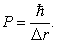(4.1)

In a gravity field, particles can not fall into empty hole. Particles can only infinity close to the empty hole. The minimum distance between a particle and an empty hole is the radius of empty hole. So, in a gravity field, the maximum momentum of a particle is (4.2).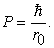(4.2)

So its maximum kinetic energy is (4.3).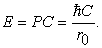(4.3)

So, in the process of empty holes merging, as the radius of the large hole increases, the kinetic energy of the small empty hole decreases. So, the formula of the kinetic energy of small empty hole is (4.4).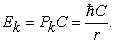(4.4)

In (4.4), r is the radius of the large empty hole.

So, in the large empty hole, the total kinetic energy of small empty holes is (4.5).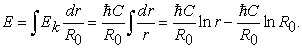(4.5)

The lower limit of the integral is the radius of the small empty hole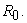The total kinetic energy is the thermodynamic internal energy of large empty hole.

Obviously, in the large empty hole, the arrangement mode of small empty holes is fixed. There is only one arrangement mode. Small empty holes can only be stacked one by one. Therefore, if there is no other degree of freedom of the small empty hole, the number of microscopic states of the large empty hole is 1. If the small holes have other degrees of freedom, obviously, the total number of microscopic states of the large empty hole is (4.6).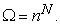(4.6)

There, n is the degree of freedom of the small empty hole, and N is number of small empty holes contained in large empty hole. For example, if small empty hole has spin property, the spin is 1, or the spin is 1/2, so the degree of freedom of the small empty hole is 2. So the total number of microscopic states of the large empty hole is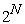.

So, the entropy of the large empty hole is (4.7). This is a very obvious result.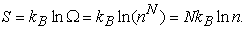(4.7)

The total number of small empty holes can be calculated directly by (4.8).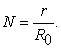(4.8)

So, the entropy of the large empty hole is (4.9).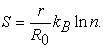(4.9)

So, the temperature of the large empty hole is (4.10).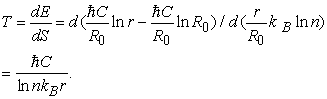(4.10)

Formula (4.10) is same with Hawking temperature formula of black hole 3. The two formula only differ by one constant ln(n). The temperature of empty hole is inversely proportional to the radius of the empty hole. The radius is larger, the temperature is smaller.

In fact, the internal energy and the entropy are theoretical calculations, and cannot be measured physically. Only the temperature can be measured physically. If we measure its temperature, the physical properties of the empty hole and the physical properties of the black hole in the General Relativity are completely equivalent.

In the derivation process to the empty hole temperature, we introduced a model of the average radius of small empty hole. According to this model, the entropy and temperature of the empty hole can be calculated.

The large empty hole is merged by many small empty holes. From (4.9), we can see that the degree of freedom of small empty hole has an important role in determining the value of the entropy of the large empty hole. Most of the particles have at least spin, with a minimum of 2 degrees of freedom. Therefore, the empty hole can be guaranteed to have the entropy, thus has the temperature.

If a large empty hole is formed by merging of multiple kind particles. Different kind particles have different degrees of freedom. One particle is one empty hole. Then, small empty holes can be grouped for calculation. The total number of microscopic states of a large empty hole is the product of the total number of microscopic states of each group. For example, there are small empty holes of different degrees of freedom such as n1, n2, n3, etc., and the number of small empty holes in each group is N1, N2, N3, and the like. Then, in such a large empty hole, the total number of microscopic states is (4.11). This is an obvious result. The entropy of a large empty hole is the sum of the entropies of each group.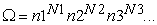(4.11)

The empty hole entropy is determined by (4.9). It can be seen that the empty hole entropy is proportional to the radius of the empty hole. Because the process of empty hole merging is actually based on the synthetic rule of gravitational mass. Without the principle of synthesis of gravitational mass, empty hole cannot be merged. Gravitational mass is linearly cumulative. Therefore, in the process of the small holes merging into large empty hole, the radius of large empty hole is also linearly accumulated. Therefore, the number of small empty holes is also linearly accumulated.

This conclusion relies on a merging model of the average radius of small empty holes. Whether the real gravitational empty hole is in conformity with such a merging model, it is not certain. There may be some conformity, and some may not. This topic is worth further research.

### 5. Derive out the Formula of Black Hole Entropy

In addition to above merging model, there is another special merging model. From this merging model, we can derive out the formula of black hole entropy in General Relativity.

To a small empty hole, it is merged into a large empty hole. The radius of the large empty hole is r. We suppose that the rest mass of the small empty hole meets a condition. The condition is (5.1).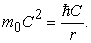(5.1)

The physical meaning of this condition is that the kinetic energy of the small empty hole is equal to its rest mass when a small empty hole is close to a large empty hole. This condition is just an idealized condition. In actual situations, just need the rest mass to approximate this value. The final result is only a constant difference. For example,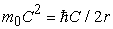, or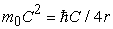. This does not have an impact on the derivation process.

The source of this condition is in previous paper 1. When solving the gravitational quantization equation, there exists this relation formula. The quantized energy of a particle is associated with this relation formula. The quantized energy of a particle in gravity field depends on a relationship parameter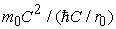. So we can think about (5.1) equation directly. Since it is not yet possible to solve the exact solution of the gravitational quantization equation, it is not possible to know the exact physical meaning of (5.1). But we already know the existence of this formula, and we know that this formula has an important significance. Only by solving the exact solution of the gravitational quantization equation can we know the exact physical meaning of this formula.

From (5.1), the radius of the small empty hole is (5.2).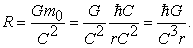(5.2)

A large empty hole is merged by many such small empty holes. So the total number of small empty holes contained in the large empty hole is (5.3).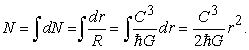(5.3)

As above, the total number of microscopic states of the large empty hole is determined by the degree of freedom of each small empty hole and the total number of small empty holes. Set n is the degree of freedom of the small empty hole, so get (5.4).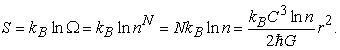(5.4)

Convert to area representation, get (5.5).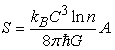(5.5)

A is the surface area of the empty hole. (5.5) is almost the same with the formula of the black hole entropy in General Relativity 3, differing only by one constant.

Because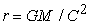, from (5.4), we get (5.6).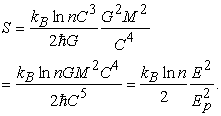(5.6)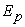is the Planck energy. From (5.5), we can get (5.7). The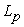is the Planck Length.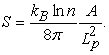(5.7)

As above, the formula of the kinetic energy of small empty hole is (5.8).(5.8)

In (5.8), r is the radius of the large empty hole.

So, in the large empty hole, the total kinetic energy of small empty holes is (5.9).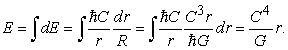(5.9)

So, the temperature of the large empty hole is (5.10).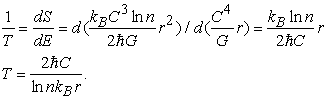(5.10)

It is same with (4.10), only one constant difference. It is same with Hawking temperature formula of black hole 3 also. These formulas only differ by one constant.

Different merging model derive out same temperature formula, only one constant difference. It all fits the theoretical expectations. Only the temperature has real physical meaning. Internal energy and entropy are only theoretical properties, and cannot be measured physically.

In this merging model, the temperature formula and the entropy formula are same with formula of black hole in General Relativity, only one constant difference.

In General Relativity, the derivation process of the black hole entropy is very complicated, and several assumptions must be made. The artificial hypothesis component is too heavy. But in empty hole merging model, only need to assume the condition (5.1), so we can derive out these formulas. Obviously, the derivation process of the empty hole entropy is simpler and more reasonable.

From the above entropy derivation process, it can be seen that the empty holes merging does not lose the entropy and does not lose the information. There is no problem of information loss. There is no contradiction with quantum mechanics.

The empty hole entropy formula is exactly the same as the black hole entropy formula, and the empty hole temperature formula is exactly the same as the black hole temperature formula. This fact needs to be further studied. The physical meaning of empty hole and black hole is completely different. Empty hole is to reject everything. Black hole is attracted to everything. Projection gravitation is a flat space-time theory. General relativity is a curved space-time theory. However, the entropy formula is the same, and the temperature formula is the same. This is a very strange situation. There is clearly an uncovered link between black hole and empty hole. There must also be an uncovered link between the projection gravitation and General Relativity.

The new projection gravitation is a nonlinear gravitation theory. Many features are different from conventional linear theory. These features need to be further studied in order to be revealed.

In above two empty holes merging model, to simplify the discussion, we have assumed a condition. The condition is that the distance between these merging empty holes is the radius of empty hole. This is an idealized condition. For the actual situation, the distance between these empty holes must be greater than the radius. For example, the distance between two holes is twice radius, or four times radius, and so on. This difference does not affect the effectiveness of the empty holes merging model at all. The final result is that the formula has a constant difference. The formula does not change.

### 6. Empty Hole Distribution

From above discussion, in the process of empty holes merging, there exists a special distribution mode. There is only one arrangement mode. Small empty holes can only be stacked one by one. There will be no jumps in the middle, and no gaps. If we think of the merging process as a filling behavior, one empty hole can only be filled in one position. From the inside to the outside, you can only fill one by one. You must fill the inside at first, and then can fill the outside. You can’t jump. This distribution mode is very similar to Fermi distribution in zero temperature. But in this distribution mode, the temperature of empty hole is not zero. And this distribution mode is completely unrelated to the spin of empty holes. This distribution is actually based on the synthetic rule of gravitational mass. This special distribution mode, can be seen as a new distribution mode. Its possible arrangement, there is only one. Therefore, if there is no other degree of freedom of the small empty hole, the number of microscopic states of the large empty hole is 1. So empty hole entropy is 0, S=ln1=0.

So the entropy of the large empty hole is determined by the degree of freedom of the small empty hole. If the small empty hole does not have the degree of freedom, the degree of freedom of the small empty hole is 1. If the total number of small holes is N, so the number of microscopic states of the large empty hole is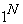. If the degree of freedom of the small empty hole is n, so the number of microscopic states of the large empty hole is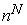. So the entropy of the large empty hole is (6.1).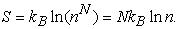(6.1)

If the n is fixed, so the entropy of the large empty hole is determined by the total number of empty holes.

We name this special distribution mode as empty hole distribution.

In the first merging model above, because we assume that each small empty hole has the same mass and radius, so the total number of small empty holes is proportional to the radius of the large empty hole, so the large empty hole entropy is proportional to the large empty hole radius. This is the physical meaning of the first merging mode.

So, we can derive out the entropy formula and temperature formula of the black hole, actually because the empty hole has this special distribution mode. This special distribution is different from other statistical distribution. It is not the Boltzmann distribution. It is not the Maxwell distribution. It is not the Fermi distribution. It is not the Bose distribution. The empty hole distribution is a new mode.

From above discussion, we can found that the black hole entropy is just a special case in empty hole. Empty hole has more thermodynamic properties than black hole.

### 7. Planck Energy and Planck Length

In above discussion, we assume the condition (5.1). We change its form, so get.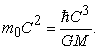That is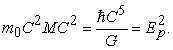(7.1)

So, we get a result of the Planck Energy. This is a very surprising result. There is a link between the condition and Planck Energy. Its physical meaning is that, the product of the rest energy of the small empty hole and the rest energy of the large empty hole must equal the square of the Planck Energy.

Let’s observe an alone empty hole. In projection gravitation, to a particle, its rest mass is associated with its wave length in 4-dimensions space.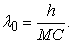(7.2)

We found a relationship between its wave length and its empty hole radius. That is (7.3). This relationship, for any empty hole, is set up.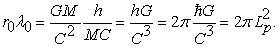(7.3)

So we get a result of the Planck Length. The wave length is associated with the 4-dimensions space. The empty hole radius is associated with the 3-dimensions. This relationship seems to indicate some association between the 4th dimension and the 3-dimensions space. This theme needs to be further studied.

So we can get a result. The Planck Energy and Planck Length have some relationship characteristic. If we observe the Planck Energy independently, we can’t found the relationship characteristic on it. The Planck Length is same yet. The two Planck constants have new physical meaning that has not yet been understood by humans. In projection gravitation theory, Planck Energy and Planck Length have a special and important role. Their physical meaning needs to be further studied.

### 8. Conclusion

In the new gravitation theory, there is empty hole. The empty hole has a radius. The empty hole is similar to the black hole in General Relativity, but they are very different. Multiple empty holes can be merged together to form a large empty hole. So the empty holes form a thermodynamic system. So the empty hole has thermodynamic properties, such as entropy and temperature. Author discusses two models of empty holes merging. From the empty holes merging model, we can derive out the entropy and temperature of empty hole. We can derive out the result that the temperature of the empty hole is inversely proportional to its radius. And we can simply derive out the formula of black hole entropy in General Relativity. The black hole entropy is just a special case in empty hole. We've only discussed two merging models, and there may be other merging models.

From the derivation process, we found a new statistical distribution mode, that is the empty hole distribution.

In the new gravitation theory, Planck Energy and Planck Length have new physical meaning. Planck Energy and Planck Length have some relationship characteristic. The two constants needs to be further studied.

The new projection gravitation theory has a lot of new properties. It needs more researchers to participate in the study, to revealing more new results.

### References

  XiaoLin Li. Projection Gravitation, a Projection Force from 5-dimensional Space-time into 4-dimensional Space-time International Journal of Physics , October 2017, Vol. 5, No. 5. http://pubs.sciepub.com/ijp/5/5/6/index.html. In article View Article  Bekenstein, Jacob D. (April 1973). “Black holes and entropy”. Physical Review D 7(8): 2333-2346. In article View Article  Bardeen, J. M.; Carter, B.; Hawking, S. W. The four laws of black hole mechanics. Comm.Math. Phys. 31 (1973), no. 2, 161-170. In article  “introduction to modern statistical mechanics”, David Chandler, ISBN-13: 978-0195042771. In article  “The Feynman Lectures on Physics (Volume I,II,III)”,R. P. Feynman,R. B. Leighton,M. Sands, ISBN 9787506272476,ISBN 9787506272483,ISBN 9787506272490. In article  Sivaram, C. (2007). “What is Special About the Planck Mass?”. https://arxiv.org/abs/0707.0058v1. In article View ArticleThis work is licensed under a Creative Commons Attribution 4.0 International License. To view a copy of this license, visit http://creativecommons.org/licenses/by/4.0/